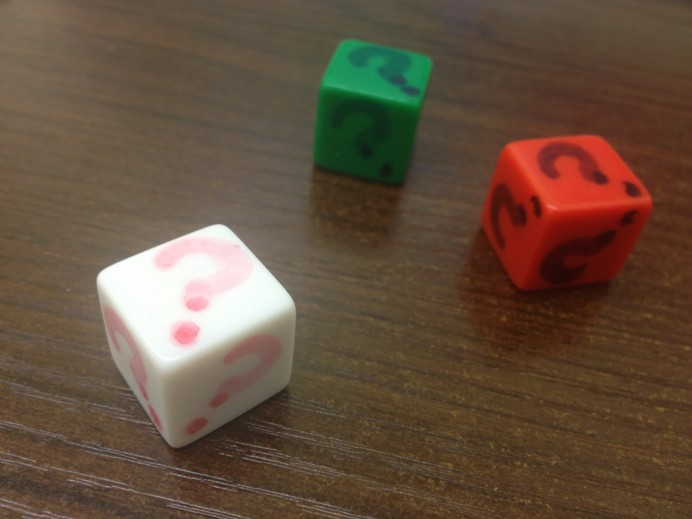Probability is a way to quantify the likelihood of an event occurring. Students explore this concept through guided interactive experiments and challenges. Some prior exposure to basic probability and familiarity with adding fractions is assumed for these programs.

Crazy Dice (Grades 5 through 12)
Once students find the probability of rolling a given sum with a pair of standard dice, they are challenged with finding a different way to number their dice to get the same probabilities. Crazy!

Evening the Odds (Grades 7 through 12)
The probability of rolling different sums with a standard pair of dice depends upon the sum in question. This activity guides students to find ways to renumber their dice so every sum appears with the same probability. But then, what sums are possible to fit into this scheme? How many different sums can be rolled with equal likelihood? The answers to these questions and more are found in this activity.

Crazier Dice (Grades 7 through 12)
If renumbering a standard pair of six-sided dice seems crazy, you might want to sit down for this activity. Students work together first to understand the probabilities encountered when rolling eight-sided dice, and then to find alternative ways to number these octahedral dice without changing those probabilities.

Back to options for educator session